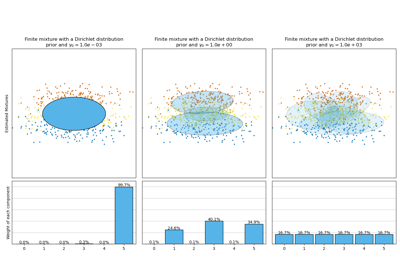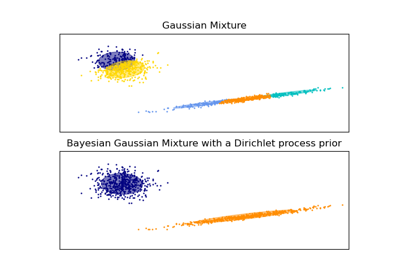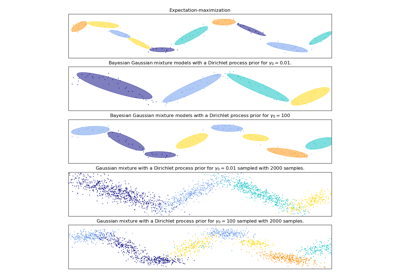# sklearn.mixture.BayesianGaussianMixture¶

class sklearn.mixture.BayesianGaussianMixture(*, n_components=1, covariance_type='full', tol=0.001, reg_covar=1e-06, max_iter=100, n_init=1, init_params='kmeans', weight_concentration_prior_type='dirichlet_process', weight_concentration_prior=None, mean_precision_prior=None, mean_prior=None, degrees_of_freedom_prior=None, covariance_prior=None, random_state=None, warm_start=False, verbose=0, verbose_interval=10)[source]

Variational Bayesian estimation of a Gaussian mixture.

This class allows to infer an approximate posterior distribution over the parameters of a Gaussian mixture distribution. The effective number of components can be inferred from the data.

This class implements two types of prior for the weights distribution: a finite mixture model with Dirichlet distribution and an infinite mixture model with the Dirichlet Process. In practice Dirichlet Process inference algorithm is approximated and uses a truncated distribution with a fixed maximum number of components (called the Stick-breaking representation). The number of components actually used almost always depends on the data.

New in version 0.18.

Read more in the User Guide.

Parameters:
n_componentsint, default=1

The number of mixture components. Depending on the data and the value of the weight_concentration_prior the model can decide to not use all the components by setting some component weights_ to values very close to zero. The number of effective components is therefore smaller than n_components.

covariance_type{‘full’, ‘tied’, ‘diag’, ‘spherical’}, default=’full’

String describing the type of covariance parameters to use. Must be one of:

'full' (each component has its own general covariance matrix),
'tied' (all components share the same general covariance matrix),
'diag' (each component has its own diagonal covariance matrix),
'spherical' (each component has its own single variance).

tolfloat, default=1e-3

The convergence threshold. EM iterations will stop when the lower bound average gain on the likelihood (of the training data with respect to the model) is below this threshold.

reg_covarfloat, default=1e-6

Non-negative regularization added to the diagonal of covariance. Allows to assure that the covariance matrices are all positive.

max_iterint, default=100

The number of EM iterations to perform.

n_initint, default=1

The number of initializations to perform. The result with the highest lower bound value on the likelihood is kept.

init_params{‘kmeans’, ‘k-means++’, ‘random’, ‘random_from_data’}, default=’kmeans’

The method used to initialize the weights, the means and the covariances. String must be one of:

‘kmeans’ : responsibilities are initialized using kmeans. ‘k-means++’ : use the k-means++ method to initialize. ‘random’ : responsibilities are initialized randomly. ‘random_from_data’ : initial means are randomly selected data points.

Changed in version v1.1: init_params now accepts ‘random_from_data’ and ‘k-means++’ as initialization methods.

weight_concentration_prior_typestr, default=’dirichlet_process’

String describing the type of the weight concentration prior. Must be one of:

'dirichlet_process' (using the Stick-breaking representation),
'dirichlet_distribution' (can favor more uniform weights).

weight_concentration_priorfloat or None, default=None

The dirichlet concentration of each component on the weight distribution (Dirichlet). This is commonly called gamma in the literature. The higher concentration puts more mass in the center and will lead to more components being active, while a lower concentration parameter will lead to more mass at the edge of the mixture weights simplex. The value of the parameter must be greater than 0. If it is None, it’s set to 1. / n_components.

mean_precision_priorfloat or None, default=None

The precision prior on the mean distribution (Gaussian). Controls the extent of where means can be placed. Larger values concentrate the cluster means around mean_prior. The value of the parameter must be greater than 0. If it is None, it is set to 1.

mean_priorarray-like, shape (n_features,), default=None

The prior on the mean distribution (Gaussian). If it is None, it is set to the mean of X.

degrees_of_freedom_priorfloat or None, default=None

The prior of the number of degrees of freedom on the covariance distributions (Wishart). If it is None, it’s set to n_features.

covariance_priorfloat or array-like, default=None

The prior on the covariance distribution (Wishart). If it is None, the emiprical covariance prior is initialized using the covariance of X. The shape depends on covariance_type:

(n_features, n_features) if 'full',
(n_features, n_features) if 'tied',
(n_features)             if 'diag',
float                    if 'spherical'

random_stateint, RandomState instance or None, default=None

Controls the random seed given to the method chosen to initialize the parameters (see init_params). In addition, it controls the generation of random samples from the fitted distribution (see the method sample). Pass an int for reproducible output across multiple function calls. See Glossary.

warm_startbool, default=False

If ‘warm_start’ is True, the solution of the last fitting is used as initialization for the next call of fit(). This can speed up convergence when fit is called several times on similar problems. See the Glossary.

verboseint, default=0

Enable verbose output. If 1 then it prints the current initialization and each iteration step. If greater than 1 then it prints also the log probability and the time needed for each step.

verbose_intervalint, default=10

Number of iteration done before the next print.

Attributes:
weights_array-like of shape (n_components,)

The weights of each mixture components.

means_array-like of shape (n_components, n_features)

The mean of each mixture component.

covariances_array-like

The covariance of each mixture component. The shape depends on covariance_type:

(n_components,)                        if 'spherical',
(n_features, n_features)               if 'tied',
(n_components, n_features)             if 'diag',
(n_components, n_features, n_features) if 'full'

precisions_array-like

The precision matrices for each component in the mixture. A precision matrix is the inverse of a covariance matrix. A covariance matrix is symmetric positive definite so the mixture of Gaussian can be equivalently parameterized by the precision matrices. Storing the precision matrices instead of the covariance matrices makes it more efficient to compute the log-likelihood of new samples at test time. The shape depends on covariance_type:

(n_components,)                        if 'spherical',
(n_features, n_features)               if 'tied',
(n_components, n_features)             if 'diag',
(n_components, n_features, n_features) if 'full'

precisions_cholesky_array-like

The cholesky decomposition of the precision matrices of each mixture component. A precision matrix is the inverse of a covariance matrix. A covariance matrix is symmetric positive definite so the mixture of Gaussian can be equivalently parameterized by the precision matrices. Storing the precision matrices instead of the covariance matrices makes it more efficient to compute the log-likelihood of new samples at test time. The shape depends on covariance_type:

(n_components,)                        if 'spherical',
(n_features, n_features)               if 'tied',
(n_components, n_features)             if 'diag',
(n_components, n_features, n_features) if 'full'

converged_bool

True when convergence was reached in fit(), False otherwise.

n_iter_int

Number of step used by the best fit of inference to reach the convergence.

lower_bound_float

Lower bound value on the model evidence (of the training data) of the best fit of inference.

weight_concentration_prior_tuple or float

The dirichlet concentration of each component on the weight distribution (Dirichlet). The type depends on weight_concentration_prior_type:

(float, float) if 'dirichlet_process' (Beta parameters),
float          if 'dirichlet_distribution' (Dirichlet parameters).


The higher concentration puts more mass in the center and will lead to more components being active, while a lower concentration parameter will lead to more mass at the edge of the simplex.

weight_concentration_array-like of shape (n_components,)

The dirichlet concentration of each component on the weight distribution (Dirichlet).

mean_precision_prior_float

The precision prior on the mean distribution (Gaussian). Controls the extent of where means can be placed. Larger values concentrate the cluster means around mean_prior. If mean_precision_prior is set to None, mean_precision_prior_ is set to 1.

mean_precision_array-like of shape (n_components,)

The precision of each components on the mean distribution (Gaussian).

mean_prior_array-like of shape (n_features,)

The prior on the mean distribution (Gaussian).

degrees_of_freedom_prior_float

The prior of the number of degrees of freedom on the covariance distributions (Wishart).

degrees_of_freedom_array-like of shape (n_components,)

The number of degrees of freedom of each components in the model.

covariance_prior_float or array-like

The prior on the covariance distribution (Wishart). The shape depends on covariance_type:

(n_features, n_features) if 'full',
(n_features, n_features) if 'tied',
(n_features)             if 'diag',
float                    if 'spherical'

n_features_in_int

Number of features seen during fit.

New in version 0.24.

feature_names_in_ndarray of shape (n_features_in_,)

Names of features seen during fit. Defined only when X has feature names that are all strings.

New in version 1.0.

GaussianMixture

Finite Gaussian mixture fit with EM.

References

Examples

>>> import numpy as np
>>> from sklearn.mixture import BayesianGaussianMixture
>>> X = np.array([[1, 2], [1, 4], [1, 0], [4, 2], [12, 4], [10, 7]])
>>> bgm = BayesianGaussianMixture(n_components=2, random_state=42).fit(X)
>>> bgm.means_
array([[2.49... , 2.29...],
[8.45..., 4.52... ]])
>>> bgm.predict([[0, 0], [9, 3]])
array([0, 1])


Methods

 fit(X[, y]) Estimate model parameters with the EM algorithm. fit_predict(X[, y]) Estimate model parameters using X and predict the labels for X. get_params([deep]) Get parameters for this estimator. Predict the labels for the data samples in X using trained model. Evaluate the components' density for each sample. sample([n_samples]) Generate random samples from the fitted Gaussian distribution. score(X[, y]) Compute the per-sample average log-likelihood of the given data X. Compute the log-likelihood of each sample. set_params(**params) Set the parameters of this estimator.
fit(X, y=None)[source]

Estimate model parameters with the EM algorithm.

The method fits the model n_init times and sets the parameters with which the model has the largest likelihood or lower bound. Within each trial, the method iterates between E-step and M-step for max_iter times until the change of likelihood or lower bound is less than tol, otherwise, a ConvergenceWarning is raised. If warm_start is True, then n_init is ignored and a single initialization is performed upon the first call. Upon consecutive calls, training starts where it left off.

Parameters:
Xarray-like of shape (n_samples, n_features)

List of n_features-dimensional data points. Each row corresponds to a single data point.

yIgnored

Not used, present for API consistency by convention.

Returns:
selfobject

The fitted mixture.

fit_predict(X, y=None)[source]

Estimate model parameters using X and predict the labels for X.

The method fits the model n_init times and sets the parameters with which the model has the largest likelihood or lower bound. Within each trial, the method iterates between E-step and M-step for max_iter times until the change of likelihood or lower bound is less than tol, otherwise, a ConvergenceWarning is raised. After fitting, it predicts the most probable label for the input data points.

New in version 0.20.

Parameters:
Xarray-like of shape (n_samples, n_features)

List of n_features-dimensional data points. Each row corresponds to a single data point.

yIgnored

Not used, present for API consistency by convention.

Returns:
labelsarray, shape (n_samples,)

Component labels.

get_params(deep=True)[source]

Get parameters for this estimator.

Parameters:
deepbool, default=True

If True, will return the parameters for this estimator and contained subobjects that are estimators.

Returns:
paramsdict

Parameter names mapped to their values.

predict(X)[source]

Predict the labels for the data samples in X using trained model.

Parameters:
Xarray-like of shape (n_samples, n_features)

List of n_features-dimensional data points. Each row corresponds to a single data point.

Returns:
labelsarray, shape (n_samples,)

Component labels.

predict_proba(X)[source]

Evaluate the components’ density for each sample.

Parameters:
Xarray-like of shape (n_samples, n_features)

List of n_features-dimensional data points. Each row corresponds to a single data point.

Returns:
resparray, shape (n_samples, n_components)

Density of each Gaussian component for each sample in X.

sample(n_samples=1)[source]

Generate random samples from the fitted Gaussian distribution.

Parameters:
n_samplesint, default=1

Number of samples to generate.

Returns:
Xarray, shape (n_samples, n_features)

Randomly generated sample.

yarray, shape (nsamples,)

Component labels.

score(X, y=None)[source]

Compute the per-sample average log-likelihood of the given data X.

Parameters:
Xarray-like of shape (n_samples, n_dimensions)

List of n_features-dimensional data points. Each row corresponds to a single data point.

yIgnored

Not used, present for API consistency by convention.

Returns:
log_likelihoodfloat

Log-likelihood of X under the Gaussian mixture model.

score_samples(X)[source]

Compute the log-likelihood of each sample.

Parameters:
Xarray-like of shape (n_samples, n_features)

List of n_features-dimensional data points. Each row corresponds to a single data point.

Returns:
log_probarray, shape (n_samples,)

Log-likelihood of each sample in X under the current model.

set_params(**params)[source]

Set the parameters of this estimator.

The method works on simple estimators as well as on nested objects (such as Pipeline). The latter have parameters of the form <component>__<parameter> so that it’s possible to update each component of a nested object.

Parameters:
**paramsdict

Estimator parameters.

Returns:
selfestimator instance

Estimator instance.

## Examples using sklearn.mixture.BayesianGaussianMixture¶Concentration Prior Type Analysis of Variation Bayesian Gaussian Mixture

Concentration Prior Type Analysis of Variation Bayesian Gaussian MixtureGaussian Mixture Model Ellipsoids

Gaussian Mixture Model EllipsoidsGaussian Mixture Model Sine Curve

Gaussian Mixture Model Sine Curve